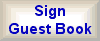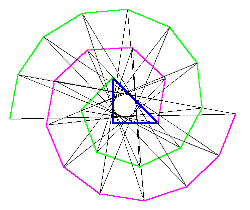What's that angular spiral all about, anyway?The Theorem of Pythagoras is one of the most famous geometrical discoveries of all time. The idea that a2 + b2 = c2, where a, b, and c are the two orthogonal sides and the hypotenuse, respectively, of a right-angled triangle, serves as the basis of the most common metric, or norm, used in the mathematics of vector spaces. It even carries over into the space-time geometry of special relativity, in the form of the Minkowski metric. The figure below was constructed by starting with an isosceles right triangle, in which the two equal sides may be considered of unit length, and the length of the hypotenuse is equal to Call this triangle ABC, with A=B=1. Then at point b (opposite side B) construct a line segment of length one that is perpendicular to side C, and connect the far end of that line segment D to point a on the original triangle. The resulting line segment E must have a length equal to Continuing in this fashion the nested spiral pattern emerges. At each step we add a new side of length one perpendicular to the hypotenuse of the last triangle we constructed, then connect it to the other end of that same hypotenuse. The lengths of the longest sides of these intertwined triangles form the sequenceIn the graphic image above, the first (isosceles) right triangle is colored blue. The green line segments are the sides of unit length in the triangles with a hypotenuse equal to the square root of some odd number, and the magenta line segments are the sides of unit length in those triangles with a hypotenuse equal to the square root of an even number. I got interested in this angular spiral because of Pell's equation. But that's a story for another day. Incidentally, the "circle" that seems to be emerging near the center of the spiral (actually, the envelope formed by the long sides of successive triangles) intuitively has a diameter equal to ½ unit. Do you see why?